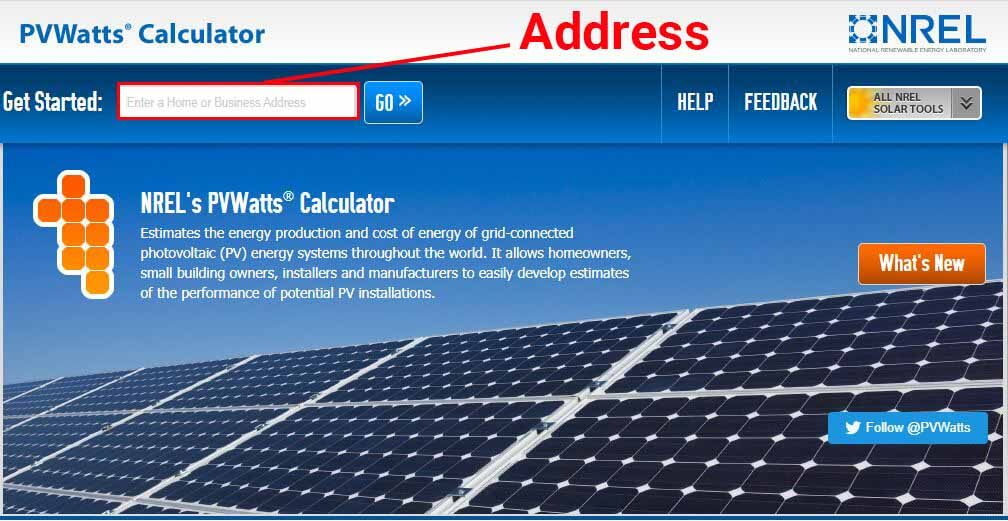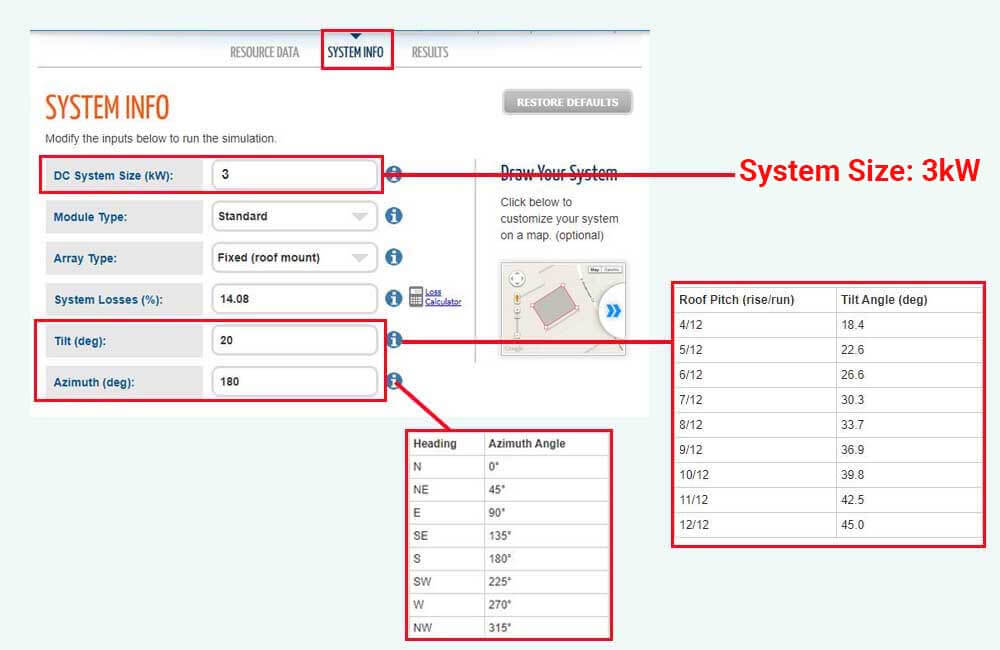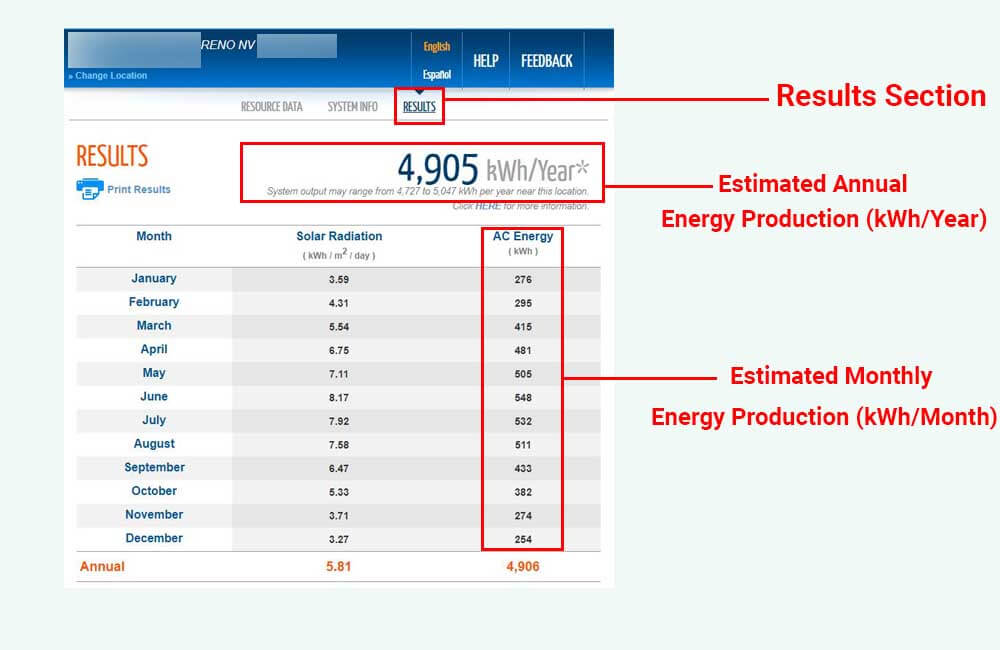# How much power does a 3kw solar system produce?

Unlike Energy, which is accumulative and is measured in Watt-hours (Wh) or kiloWatt-hours (kWh), Power is instantaneous and is measured in Watts (W) or kiloWatts (kW). Power measures the rate at which Energy is being generated.

For example, a 3kW (3000 Watt) solar system is capable of producing 3000 Watts of power, or even more, under the right conditions. If a 3kW solar system constantly produces 3000 Watts of power for one hour, it will have generated 3000 Watt-hours of energy by the end of that hour.

However, the actual amount of power that a system of this size produces is not constant and will fluctuate during the day depending on how much sunlight is getting to the solar panels.

So, what really matters at the end of the day, is not the amount of power that a 3kW system produces, but, the average amount of energy that it generates on a daily basis.

In other words, the important question to ask is not “How much power does a 3kW solar system produce?”, but “On average, how much energy does a 3kW solar system produce?”.

In this article, I will discuss the energy production of a 3kW system, and the factors that influence it, and I will explain how you can determine it.

## How much energy does a 3kw solar system produce?

The amount of energy that a 3kW solar system produces will mainly depend on its location, seasons, and the configuration of the system such as tilt angle (Elevation) and orientation (Azimuth).

However, in general, a 3kW solar system would on average produce around 12kWh (kiloWatt-hours) of energy per day, which amounts to about 360 kWh of energy per month, and 4400 kWh of energy per year.

Depending on where the system is located, which time of year it is, the tilt angle of the solar panels, and the direction they’re facing, in a single day, a 3kW solar system can generate as much as 20 kWh or as little as 3 kWh of energy.

Here’s a table that compares the average daily energy production of a 3kW solar system (in kWh) in different states around the U.S., in the months of June and December:

Please note that the figures provided in the table are based on the assumption that the solar panels in the 3kW system are tilted at an angle of 20 degrees, and are facing South.

Also, it is important to note that even in the same state, the average daily energy production of a 3kW solar system could differ from city to city.

The good news is, you can actually determine the average daily energy production of a 3kW system in your exact location. This can be done using the PVWatts Calculator by NREL (National Renewable Energy Laboratory), which is totally free.

Using historical solar irradiation data, the PVWatts Calculator can estimate the monthly and yearly energy production of a solar system based on:

• The exact location of the system.
• The angle at which the solar panels are tilted.
• The orientation of the solar panels.

To access this data, follow these steps:

### Step 1: Submit your location### Step 2: Describe your system

Once you’ve entered your location, go to the “System Info” section of the tool.As shown in the image above, you’ll find 6 fields in the “System Info” section of the tool. I recommend you leave the Module Type, Array Type, and System Losses fields to their default values, and modify the values in the following fields:

DC System Size (kW):

In this field, enter the Power rating of your solar system in kiloWatt (kW). In our case, the system is rated at 3kW.

Tilt (deg):

The angle at which your solar panels are going to be tilted, or their elevation angle, will have a direct impact on how much sunlight they receive, and therefore, on how much power and energy they generate. Make sure to enter the correct tilt angle to get accurate estimates.

For example, if the solar panels are going to be installed on a flat surface such as that of an RV, the tilt angle is going to be 0 degrees.

If the solar panels are going to be installed on a sloped roof, you can click on the information “i” icon next to the field to determine the equivalent of your roof pitch in degrees. For example, if the roof section has a 6/12 roof pitch, the solar panels are going to be tilted at a 26.6-degree angle.

If you don’t exactly know the slope of the roof section, you can use a smartphone app such as Measure for iOS or Bubble Level for Android to determine the tilt angle of that roof section.

Azimuth (deg):

Azimuth represents the Heading or the orientation of the solar panels. A 0-degree Azimuth would mean that the solar panels are facing North, a 90-degree Azimuth would mean the solar panels are facing East, and a 180-degree Azimuth would mean that the solar panels are facing South.

The orientation of the solar panels will also influence the amount of sunlight that they receive, and the amount of energy that they produce.

In the U.S. (or locations north of the Equator in general), solar panels are commonly oriented to face South, because that’s the heading that would result in optimal energy production. In Australia (or locations south of the Equator), the optimal heading is North.

If the solar panels are going to be installed on a flat surface (0-degree tilt angle), the Azimuth angle will not be of much influence. However, if it’s a roof installation, and the roof is sloped, make sure to enter the correct Azimuth angle to get accurate estimates.

If you don’t know the exact heading of the roof section on which your 3kW system is going to be installed, you can use a compass app such as Commander Compass Go for iOS or Azimuth Compass for Android to determine its Azimuth.

Once you submit the size of the system (3kW), its Tilt Angle, and Azimuth, the last step is to go to the “Results” section of the PVWatts Calculator to get your estimates.

### Step 3: Read and understand the results

To understand this better, I’m going to use the following example:

• DC System Size: 3kW.
• Tilt: I’ll assume the system is roof-mounted with a 5/12 roof pitch, which is equivalent to a tilt angle of 22.6 degrees.
• Azimuth: I’ll assume that the only unshaded roof section available is facing South-West, this equates to an Azimuth of 225 degrees.

In the “Results” tab, the calculator provided the following data:The PVWatts Calculator has estimated that a 3kW solar system in that particular location in Reno, NV, that is titled at an angle of 22.6 degrees, and that is facing South-West, would on average produce 4905 kWh of energy per year.

In the table, you can also see energy production estimates for each month, which you can divide by the number of days in that particular month (28, 30, or 31) to determine the average daily energy production in that specific month.

For example, while the 3kW solar system would only produce about 254 kWh of energy in December, which translates to 8.2 kWh of energy per day, the 3kW system would produce around 505 kWh of energy in May, which is equivalent to about 16.3 kWh/day (almost double the energy production in December).

Once you’ve estimated the amount of energy that the 3kW system is going to produce, the next section will help you put this amount of energy into perspective.

## What can a 3kw solar system run?

As explained above, a 3kW solar system produces around 12 kWh of energy per day, but what can you run on this amount of power?

For perspective, the U.S. Energy Information Administration (EIA) estimates that the average American household consumes about 29-30 kWh of energy per day. This translates to about 850-900 kWh per month or 10600-11000 kWh per year.

In other words, a 3kW solar system would only be able to offset about 40% of the energy consumption of the average U.S. household.

However, for grid-tied systems, the solar system doesn’t really have to offset 100% of your energy consumption for it to work. If your energy consumption is similar to that of the average American household, and you’re planning on installing a grid-tied 3kW solar system, it will still be able to reduce your energy bill by around 40%.

If you’re planning on installing a 3 kW off-grid solar system, depending on which appliances you plan on running and how often or how long you use these appliances, the system might be able to offset 100% of your energy consumption.

To provide perspective, the following table provides some common household appliance and their hourly energy consumption (Wh/hour):

Related topics:

How much electricity does an air conditioner use?

How much power does a TV use?

Please note that the figures in the table are rough estimates, the actual energy consumption of your appliances will depend on the specific model. The best way to determine the energy consumption of an appliance is to actually measure it using an electricity monitoring device.

## How many solar panels do I need for a 3kw system?

In general, you would need between 8 and 15 solar panels for a 3kW solar system. The exact number of solar panels that you need to make up a 3 kW solar system will depend on the Power rating (Wattage) of the solar panels you plan on using.

For example, if you use 250W solar panels, you’ll need 12 solar panels to make up 3000 Watts (3000W ÷ 250W = 12). If you use 300W solar panels, you’ll need 8 solar panels (3000W ÷ 300W = 10).

## How big is a 3kw solar system?

A 3kW solar system would require between 170 and 200 sq. ft. (16 to 18 sq. m) of roof space depending on how efficient the solar panels are. The more efficient the solar panel used in the 3kW system, the less space will be needed.

Related:

How big is a solar panel?

How many solar panels will fit on my roof?

## How many batteries for a 3kw solar system

As mentioned above, a 3kW solar system will produce around 12 kWh (or 12000 Wh) of energy per day. To be able to store and access that amount of energy, you would need – at least – 10 batteries rated at 12V-100Ah, 5 batteries rated at 24V-100Ah, or 3 batteries rated at 48V-100Ah.

However, solar battery banks should not be sized based on the potential energy production of the solar array but based on your daily energy consumption.

In addition to your daily energy consumption, other factors such as the Depth Of Discharge and days of autonomy should be taken into consideration when calculating the required battery capacity.

Read more on this subject here: How to calculate battery capacity for a solar system?